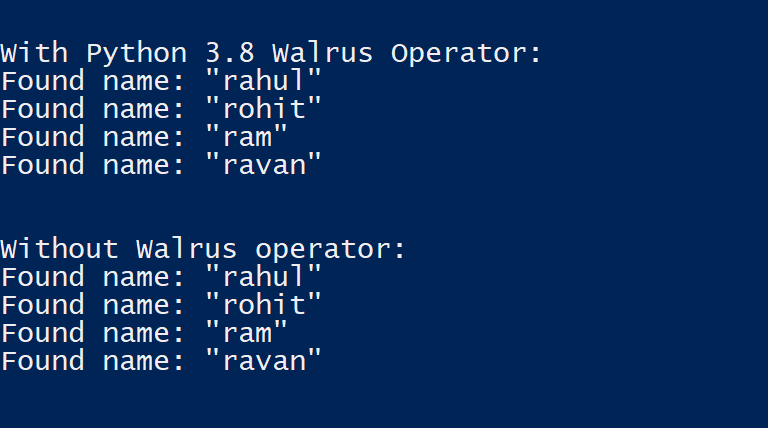# Walrus Operator in Python 3.8

• Difficulty Level : Easy
• Last Updated : 26 Aug, 2019

Python 3.8 is still in development. But many alpha versions have been released. One of the latest features in Python 3.8 is the Walrus Operator. In this article, we’re going to discuss the Walrus operator and explain it with an example.

Introduction

Walrus-operator is another name for assignment expressions. According to the official documentation, it is a way to assign to variables within an expression using the notation NAME := expr. The Assignment expressions allow a value to be assigned to a variable, even a variable that doesn’t exist yet, in the context of expression rather than as a stand-alone statement.

Code :

 `a ``=` `[``1``, ``2``, ``3``, ``4``]``if` `(n :``=` `len``(a)) > ``3``:``    ``print``(f``"List is too long ({n} elements, expected <= 3)"``)`

Output :Here’s instead of using “len(a)” in two places we have assigned it to a variable called “n”, which can be used later. This helps us to resolve code duplication and improves readability.

Example –

Let’s try to understand Assignment Expressions more clearly with the help of an example using both Python 3.7 and Python 3.8. Here we have a list of dictionaries called “sample_data”, which contains the userId, name and a boolean called completed.

 `sample_data ``=` `[``    ``{``"userId"``: ``1``,  ``"name"``: ``"rahul"``, ``"completed"``: ``False``},``    ``{``"userId"``: ``1``, ``"name"``: ``"rohit"``, ``"completed"``: ``False``},``    ``{``"userId"``: ``1``,  ``"name"``: ``"ram"``, ``"completed"``: ``False``},``    ``{``"userId"``: ``1``,  ``"name"``: ``"ravan"``, ``"completed"``: ``True``}``]`` ` `print``(``"With Python 3.8 Walrus Operator:"``) ``for` `entry ``in` `sample_data: ``    ``if` `name :``=` `entry.get(``"name"``):``        ``print``(f``'Found name: "{name}"'``)`` ` `print``(``"Without Walrus operator:"``)``for` `entry ``in` `sample_data:``    ``name ``=` `entry.get(``"name"``)``    ``if` `name:``        ``print``(f``'Found name: "{name}"'``)`

Output:My Personal Notes arrow_drop_up